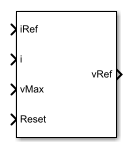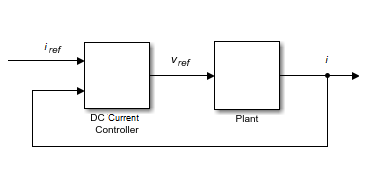# DC Current Controller

Discrete-time DC current PI control with integral anti-windup

•Libraries:
Simscape / Electrical / Control / General Machine Control

## Description

The DC Current Controller block implements a discrete-time proportional-integral (PI) DC voltage controller. The block can implement zero cancellation in the feedforward path. To avoid saturation of the integral gain, the block can implement anti-windup gain.### Equations

The equation that the DC Current Controller block uses to calculate the reference voltage is

`${v}_{ref}=\left({K}_{p}+{K}_{i}\frac{{T}_{s}z}{z-1}\right)\left({i}_{ref}-i\right),$`

where:

• vref is the reference voltage.

• Kp is the proportional gain.

• Ki is the integral gain.

• Ts is the sample time.

• iref is the reference current.

• i is the measured current.

The PI control calculation yields a zero in the closed-loop transfer function. To cancel the zero, the block uses this discrete-time zero-cancellation transfer function:

`${G}_{ZC}\left(z\right)=\frac{\frac{{T}_{s}{K}_{i}}{{K}_{p}}}{z+\left(\frac{{T}_{s}-\frac{{K}_{p}}{{K}_{i}}}{\frac{{K}_{p}}{{K}_{i}}}\right)}.$`

To avoid saturation of the integrator output, the block uses an anti-windup mechanism. The integrator gain is then equal to

`${K}_{i}+{K}_{aw}\left({v}_{ref\text{_}sat}-{v}_{ref\text{_}unsat}\right),$`

where:

• Kaw is the anti-windup gain.

• vref_sat is the saturated reference voltage signal, which the block calculates as ${v}_{ref\text{_}sat}=\text{min}\left(\mathrm{max}\left({v}_{ref\text{_}unsat},{v}_{min}\right),{v}_{max}\right),$

where:

• vref_unsat is the unsaturated reference voltage signal.

• vmin is the lower limit for the output voltage. For positive voltage only, ${v}_{min}=0$. For positive and negative voltage, ${v}_{min}=-{v}_{max}$

• vmax is the upper limit for the output voltage.

## Ports

### Input

expand all

Desired output current for the plant.

Data Types: `single` | `double`

Measured current for the plant.

Data Types: `single` | `double`

Maximum DC output voltage for the plant.

Data Types: `single` | `double`

External reset signal (rising edge) for the integrator.

Data Types: `single` | `double`

### Output

expand all

Desired DC output voltage for the plant.

Data Types: `single` | `double`

## Parameters

expand all

Proportional gain, Kp, of the controller.

Integral gain, Ki, of the controller.

Anti-windup gain, Kaw, of the controller.

Allow for both positive and negative DC voltage output or limit the output to positive DC voltage.

Time, in s, between consecutive block executions. During execution, the block produces outputs and, if appropriate, updates its internal state. For more information, see What Is Sample Time? and Specify Sample Time.

If this block is inside a triggered subsystem, inherit the sample time by setting this parameter to `-1`. If this block is in a continuous variable-step model, specify the sample time explicitly using a positive scalar.

#### Dependencies

If you set Sample time (-1 for inherited) to `-1` and select the Enable zero cancellation option, the Discretization sample time parameter becomes visible.

Time, in s, between consecutive discretizations. Discretization is required for zero cancellation.

#### Dependencies

This parameter is only visible when both these conditions are met:

• is set to `-1`.

• Enable zero cancellation is selected.

Option to use zero cancellation on the feedforward path.

#### Dependencies

If you select the Enable zero cancellation option and set Sample time (-1 for inherited) to `-1`, the Discretization sample time parameter becomes visible.

## Version History

Introduced in R2018a